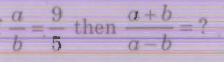# Group 2 2015 July GT TNPSC Question Paper

## Group 2 2015 July GT TNPSC Questions

41.

Find the range of the following data :

25, 67, 78, 43, 21, 17, 49, 54, 76, 92, 20, 45, 86, 37, 35

A.

78

B.

75

C.

92

D.

86

B. 75

42.
A is B's sister, C is B's Mother, D is C's father E is D's mother. Then, A is the of D
A.
Grand mother
B.
Grand father
C.
Daughter
D.
Grand daughter
D. Grand daughter
43.
A is richer than B, C is richer than A, D is richer than C. E is the richest of all. If they are made to sit in the above degree of richness, who will have the central position?
A.
A
B.
B
C.
C
D.
D
C. C
44.
A hollow cylindrical iron pipe of length 35 cm. Its outer and inner diameters are 10 cm and 8 cm respectively. Find the weight of the pipe if 1 cu.cm of iron weighs 7 gm.
A.
6.93 kg
B.
9.90 kg
C.
7.53 kg
D.
7.93 kg
A. 6.93 kg
45.

Find out the missing term in the given alphabet series :

AZ, GT, MN,____, YB

A.

JH

B.

SH

C.

SK

D.

TS

B. SH

46.
7 men can complete a work in 52 days. In how many days will 13 men finish the same work?
A.
20 days
B.
13 days
C.
7 days
D.
28 days
D. 28 days
47.

IfA.

3/7

B.

7/3

C.

2/7

D.

7/2

D. 7/2

48.
Find the L.C.M of 45,4-81,412 and 47
A.
412
B.
4
C.
42
D.
4-2
A. 412
49.

Find the missing number in the following :

4242, 4254, 4230, 4266, 4218, 4278, _________

A.

4264

B.

4272

C.

4228

D.

4206

D. 4206

50.
The average of 4 values is 20 and when a quantity is added to each value the average is 22. Find the quantity.
A.
1
B.
2
C.
3
D.
4